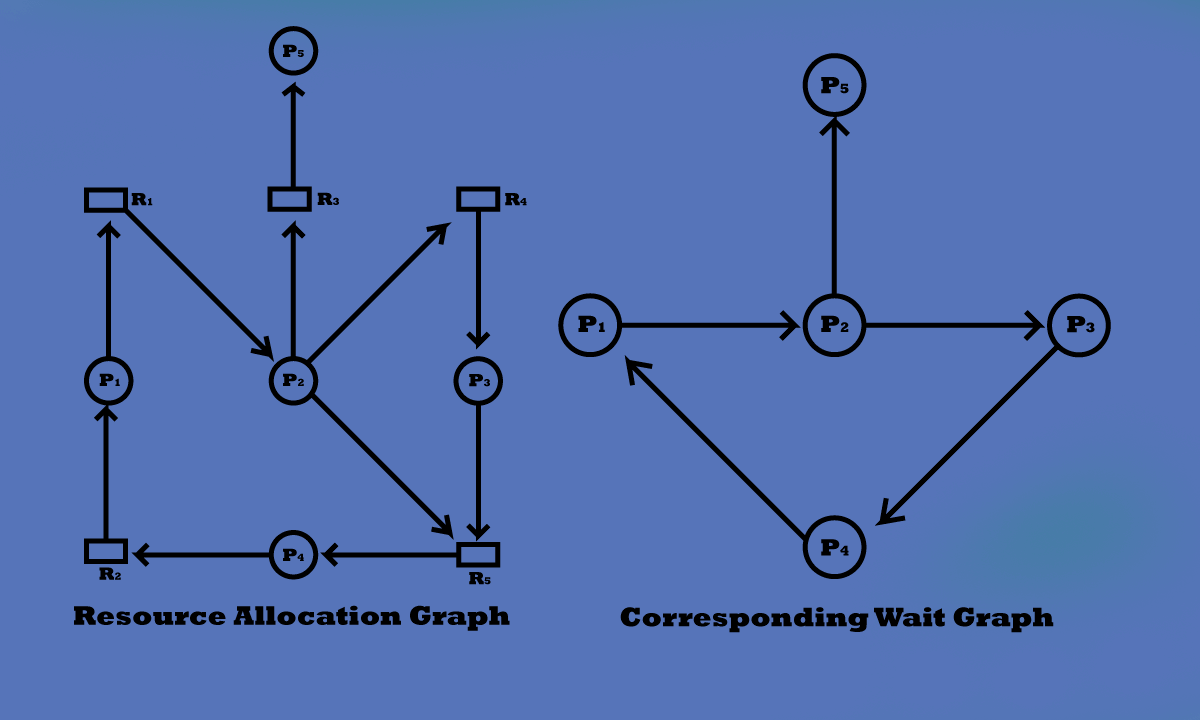Deadlock problems can only become more common, given current trends, including larger numbers of processes, multithreaded programs, many more resources within a system, and emphasis on long-lived file and database servers rather than batch systems. So, you need to know How can you detect deadlock in your system & recover a system from deadlock?

There are two algorithms for deadlock detection. They are;

1. One for the system with a single instance of each resource type.
2. Other for the system with multiple instances of a resource type.

The system with a single instance of each resource type: this algorithm uses a variant of the resource allocation graph, called the wait-for graph.

In this graph, there is an edge from process Pi to process Pj, indicating that process Pi is waiting for process Pj to release a resource that Pi needs. Also, an edge P→ Pj exists in a wait-for graph. If and only if the corresponding resource-allocation graph contains two edges P→ Rq and R→ Pj for some resource Rq.

A deadlock exists if and only if the wait-for contains a cycle.

Let us consider the following wait-for graph obtained from the corresponding resource-allocation as also shown:Figure: System with Single Instance of each Resource Type

Here, a deadlock is occurred because there exist cycles in the wait-for graph.

The system with multiple instances of a resource type: This deadlock detection algorithm employs several time-varying data structures as follows:

• Available: A vector of length m indicates the number of resources of each type.
• Allocation: An n x m matrix defines the number of resources of each type currently allocated to each process.
• Request: an n x m matrix indicates the current request for each process. If request [i, j] = k, then process Pi is requesting k more instances of resource type Rj.

This algorithm investigates every possible allocation sequence for the process that remains to be completed. The algorithm is described below:

1. Let Work and Finish be vectors of length m and n respectively. Initialize Work = Available. For i = 0,1,2,3,…..n-1, if Allocationi ≠ 0, then Finish [i] = false. Otherwise Finish [i] = true.

2. Find an index i such that both

Finish[i] == false.

Requesti ≤ Work.

If no such i exists, go to step 4.

3. Work = Work + Allocationi

Finish[i] = true.

Go to step 2

4. If Finish [i] = false for some i, 0 ≤ i ≤ n, then the system is in a deadlock state.

Moreover, if Finish [i] == false, then process Pi is deadlock.

How do you recover a system from deadlock?

When a deadlock is detected, the system can recover from the deadlock automatically. There are two options breaking a deadlock:

Aborting Process: to eliminate deadlocks by aborting a process, we use one of two methods, the system reclaims all resources allocated to the terminated processes.